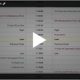# Hedging and Risk Management for Equity Index Futures

The idea behind risk management is to ensure you will not incur losses greater than your risk tolerance. In practice, it means balancing risk versus reward and then making an informed investment decision.

A key component of risk management is hedging. Hedging is like insurance; you hope things go as planned, but if they do not, then what? Hedging can provide a means of protection for unplanned or negative events.

Where Notional Value Comes In

There is an old expression, “you can’t manage what you can’t measure.” Risk managers and traders can use Equity Index futures to help manage their equity index risk. It begins with understanding the notional value of the Equity Index futures contract. The notional value of a futures contract represents that contract’s financial equivalent value. For Equity Index futures, it is calculated:

Notional value = price of futures contract x contract multiplier.

In the case of the E-mini S&P 500 futures that have a fixed multiplier of \$50, it might look like this: 2185.00 (futures price) x \$50 = \$109,250 per contract. The notional value represents the financial value of the contract at that price level.

Using Notional Value as Part of a Hedging Strategy

Traders use notional value to compare the current value of the futures price to other futures contracts or highly correlated physical positions. When calculating a hedge ratio, traders and risk managers would want to compare the value of the instrument of portfolio at risk versus the relative value of the futures contract.

EXAMPLE

For example, assume the E-mini S&P 500 futures are priced at \$2185.00, which results in a notional value of \$109,250. Now consider a portfolio manager with a \$10 million S&P 500 equity risk position. Suppose she wants to reduce her exposure to the S&P 500 index by 10%. She could utilize E-mini S&P 500 futures by selling futures in a ratio based on the notional value of the futures contract.

Hedge ratio = value at risk ÷ notional value of futures contract

In this case, 10% of \$10 million is \$1 million that needs to be hedged. To calculate the equivalent futures contracts needed to hedge this position, divide \$1 million by the notional value of the futures contract, which is \$109,250. This equals 9.15, or the equivalent of nine E-mini S&P 500 futures contracts.

Say the S&P 500 index goes down by 3%, from 2185.00 to 2119.50, a drop of 65.5 index points. The portfolio loses 3% or \$300,000. If the manager sold nine futures contracts, she would have gained \$29,475. This is derived as follows: 2185.00 – 2119.50 = 65.50 index points: 65.50 index points x \$50 per point x 9 contracts = \$29,475.

The portfolio manager’s net result is \$300,000 minus \$29,475 = \$270,525 or a 2.7% loss rather than 3.0%. She has effectively reduced her losses by 10% adding value to her shareholders.

How We Can Help

We trade Equity Index products providing risk-management tools that serve to assist equity portfolio managers in even the most challenging market environments. By providing deeply liquid, actionable prices on benchmark index futures and options, we can provide risk managers and traders with the tools to implement their hedging and tactical trading strategies.

If you have questions send us a message or schedule an online review .

Regards,

—————————————————————-

Privacy Notice

Disclosure